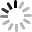# JavaScript 同步延遲 ( Promise + setTimeout )## 同步非同步``````setTimeout(function(){
console.log('A');
},1000);

setTimeout(function(){
console.log('B');
},1000);
``````

## Promise

``````function asyncFunction(value) {
return new Promise(function(resolve, reject){
if(value){
resolve(value) // 已實現，成功
}else{
reject(reason) // 有錯誤，已拒絕，失敗
}
});
}
``````

## 同步延遲

``````function delay() {
return new Promise(function (resolve, reject) {
setTimeout(function () {
resolve('我是傳下去的值');
}, 1000);
});
}

delay().then(function (value) {
console.log(value);    // '我是傳下去的值'
}).catch(function (error) {
console.log(error);
});
``````

``````setTimeout(function(){
console.log(1);  // 顯示 1
a(2);            // 呼叫 a 顯示 2
});

function a(v){
setTimeout(function(){
console.log(v);  // 延遲一秒之後顯示 2
b(3);            // 延遲一秒之後呼叫 b 來顯示 3
},1000);
}

function b(v){
setTimeout(function(){
console.log(v);    // 延遲一秒之後顯示 3
},1000);
}
``````

``````var delay = function(s){
return new Promise(function(resolve,reject){
setTimeout(resolve,s);
});
};

delay().then(function(){
console.log(1);     // 顯示 1
return delay(1000); // 延遲ㄧ秒
}).then(function(){
console.log(2);     // 顯示 2
return delay(1000); // 延遲一秒
}).then(function(){
console.log(3);     // 顯示 3
});
``````

## resolve 回傳值

``````var delay = function(r,s){
return new Promise(function(resolve,reject){
setTimeout(function(){
resolve([r,s]);
},s);
});
};

delay('a',0).then(function(v){
console.log(v,v);   // 顯示 a 0
return delay('b',1000);   // 延遲一秒之後，告訴後面的函示顯示 b 1000
}).then(function(v){
console.log(v,v);   // 顯示 b 1000
return delay('c',2000);   // 延遲兩秒之後，告訴後面的函示顯示 c 2000
}).then(function(v){
console.log(v,v);   // 顯示 c 2000
});
``````